# 2021年成人高考高起点《理数》模拟试题六

2021-03-04 15:22 江西成考网

1.已知两圆的方程为x2+y2+4x-5=0 和 x2+y2-12x-12y+23=0，那么这两个圆的位置关系是（   ）

A.相交
B.外切
C.内切
D.相离

2.已知函数f(x)为奇函数，当x∈(0，1)时，f(x)=lg(x+1)，那么，当x∈(-1，0)时，f(x)的表达式为 （   ）

A.B.C.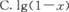D.3.直线ι的方程为x-y-2=0，它关于点(1，-4)的对称直线方程为（   ）

A.x+y-8=0
B.x-y-8=O
C.x+y+8=0
D.x-y+8=O

4.设a=(5,8),b=(2,3),c=(1,-2),则(a·b)·c等于（   ）

A.(34,-68)
B.34
C.-34
D.31

5.A.B.16(2-)
C.16(2+)
D.16

6.（   ）

A.直线
B.射线
C.线段
D.圆

7.用0，1，2，3，4，5这六个数字，可组成没有重复数字的六位数的个数是（　　）

A.120
B.600
C.714
D.720

8.A.充要条件
B.必要不充分条件
C.充分不必要条件
D.非必要亦非充分条件

9.（   ）

A.(-4，5)，(-1，1)
B.(-4，5)，(0，0)
C.(-1，2)，(0，0)
D.(-3，4)，(-1，2)

10.已知2a+b=3c，3a-6=2c，a与b的关系是（　　）

A.a=b
B.a=2b
C.a=-b
D.a=-2b

11.过点M(-3，2)，且与向量a=(-2，1)平行的直线方程是（   ）

A.x-2y+7=0
B.x+2y-1=0
C.2x+y+8=0
D.x+2y+4=O

12.“a=b”是“方程ax2+by2=1表示的曲线为圆”的（   ）

A.充分非必要条件
B.必要非充分条件
C.充分且必要条件
D.既非充分又非必要条件

13.（   ）

A.12
B.24
C.16
D.48

14.（   ）

A.B.C.D.15.（   ）

A.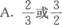B.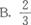C.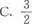D.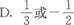16.A.B.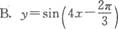C.D.17.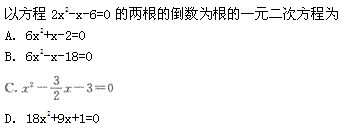（   ）

A.A
B.B
C.C
D.D

18.19.20.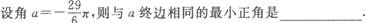21.22.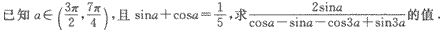23.24.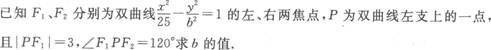25.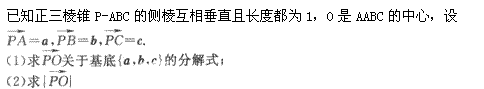186
2021年成人高考考试时间
10月24 25日+